• matlab子函数调用
千次阅读
2021-04-21 11:33:21

来源：学生作业帮 编辑：作业帮 分类：综合作业 时间：2021/03/18 18:00:53

matlab主函数如何调用子函数

我编写了一个matlab子函数是关于龙格库塔计算的

主函数内容为：

format long

i=145;

t=i*0.001;

x=zeros(2,1);

x(1,1)=35;

f=t*sin(x(1,1));

x(2,1)=Runge_Kutta(x(1,1));

子函数内容为：

function x1=Runge_Kutta(x)

%Runge-Kutta积分方法解算

T=0.01;

%设周期是0.01秒,具体的计算中再修改

f(x,t)=t*sin(x);

%先假设f(x,t)是一个这样的函数,具体函数在具体问题中再修改

x=x(1,1);

%x先假设一个值,到具体问题时再具体输入修改

K1=t*sin(x);

K2=(t+T/2)*sin(x+K1/2);

K3=(t+T/2)*sin(x+K2/2);

K4=(t+T)*sin(x+K3);

x1=x+(T/6)*(K1+2*K2+2*K3+K4);

%龙格库塔方法计算过程

在计算过程中提示出现的问题是Error in ==> diaoyong1 at 8

x(2,1)=Runge_Kutta(x(1,1));

请问在调用子程序时该怎么样修改这两个函数,

zcy_179的回答,我还有一个问题

就是这个f函数可不可以放在子程序里面,

在他的回答中放到了主程序中,如果可以该怎么设置,

function main

format long

i=145;

t=i*0.001;

x=zeros(2,1);

x(1,1)=35;

f=t*sin(x(1,1));

x(2,1)=Runge_Kutta(t,x(1,1))

%子函数内容为：

function x1=Runge_Kutta(x)

%Runge-Kutta积分方法解算

T=0.01;

%设周期是0.01秒,具体的计算中再修改

%f(x,t)=t*sin(x); %这个语句肯定有问题,不起作用,所以注销掉

%先假设f(x,t)是一个这样的函数,具体函数在具体问题中再修改

x=x(1,1);

%x先假设一个值,到具体问题时再具体输入修改

K1=t*sin(x);

K2=(t+T/2)*sin(x+K1/2);

K3=(t+T/2)*sin(x+K2/2);

K4=(t+T)*sin(x+K3);

x1=x+(T/6)*(K1+2*K2+2*K3+K4);

%龙格库塔方法计算过程

以上计算的结果为

x =

35.000000000000000

34.999399730655441

更多相关内容
• matlab子函数调用方法说明-子函数调用.pdf 今天看了一下matlab子函数的调用，发现这个资料比较好，说的比较清楚，和大家共享一下。 子函数调用.pdf 子函数调用方法
• 来源：学生作业帮 编辑：作业帮 分类：综合作业 时间：2021/03/18 18:00:53matlab主函数如何调用子函数我编写了一个matlab子函数是关于龙格库塔计算的主函数内容为：format longi=145;t=i*0.001;x=zeros(2,1);x(1,1)...

来源：学生作业帮 编辑：作业帮 分类：综合作业 时间：2021/03/18 18:00:53

matlab主函数如何调用子函数

我编写了一个matlab子函数是关于龙格库塔计算的

主函数内容为：

format long

i=145;

t=i*0.001;

x=zeros(2,1);

x(1,1)=35;

f=t*sin(x(1,1));

x(2,1)=Runge_Kutta(x(1,1));

子函数内容为：

function x1=Runge_Kutta(x)

%Runge-Kutta积分方法解算

T=0.01;

%设周期是0.01秒,具体的计算中再修改

f(x,t)=t*sin(x);

%先假设f(x,t)是一个这样的函数,具体函数在具体问题中再修改

x=x(1,1);

%x先假设一个值,到具体问题时再具体输入修改

K1=t*sin(x);

K2=(t+T/2)*sin(x+K1/2);

K3=(t+T/2)*sin(x+K2/2);

K4=(t+T)*sin(x+K3);

x1=x+(T/6)*(K1+2*K2+2*K3+K4);

%龙格库塔方法计算过程

在计算过程中提示出现的问题是Error in ==> diaoyong1 at 8

x(2,1)=Runge_Kutta(x(1,1));

请问在调用子程序时该怎么样修改这两个函数,

zcy_179的回答,我还有一个问题

就是这个f函数可不可以放在子程序里面,

在他的回答中放到了主程序中,如果可以该怎么设置,

function main

format long

i=145;

t=i*0.001;

x=zeros(2,1);

x(1,1)=35;

f=t*sin(x(1,1));

x(2,1)=Runge_Kutta(t,x(1,1))

%子函数内容为：

function x1=Runge_Kutta(x)

%Runge-Kutta积分方法解算

T=0.01;

%设周期是0.01秒,具体的计算中再修改

%f(x,t)=t*sin(x); %这个语句肯定有问题,不起作用,所以注销掉

%先假设f(x,t)是一个这样的函数,具体函数在具体问题中再修改

x=x(1,1);

%x先假设一个值,到具体问题时再具体输入修改

K1=t*sin(x);

K2=(t+T/2)*sin(x+K1/2);

K3=(t+T/2)*sin(x+K2/2);

K4=(t+T)*sin(x+K3);

x1=x+(T/6)*(K1+2*K2+2*K3+K4);

%龙格库塔方法计算过程

以上计算的结果为

x =

35.000000000000000

34.999399730655441

展开全文• matlab里怎么调用函数每一滴泪，每一道疤，是自己成长的代价，小编曾经犹豫过，葛然回首，小编不后悔。先保存你所编写的函数函数 function drawsur(surface), switch surface case 'sphere',sphere case '...

matlab里怎么调用函数每一滴泪，每一道疤，是自己成长的代价，小编曾经犹豫过，葛然回首，小编不后悔。

先保存你所编写的函数 如函数 function drawsur(surface), switch surface case 'sphere',sphere case 'cylinder',cylinder end shading interp axis equal 保存在D:\My Documents\MATLAB\li1_5_5.m 则调用该函数就在命令窗口中输入li1_5_5('sp会有那么一天，小编也会放下如今的执着和不舍，带着稍许的遗憾，过着没有你的新生活。

matlab主函数如何调用子函数？

当你没有把你编辑好的子函数放入正确的文件夹时，不管你输入的程序是否有错，当你执行时，系统都会提示“没有定义”，这个时候就是没有子函数文件放到主函数库文件夹中，所示。 后来你发现曾经讨厌吃的菜也不再那么难吃了，失败的恋情到现在也成了侃侃而谈的资本，曾经你以为遇见了人生最难过的一道坎，后来发现接下来一道比一道难过，只要翻过午夜点，你白天的经过都将成为经历，所以啊，有时要有铁了心的勇气去做一件事爱一个人，无论好坏，明天都会到来。

就算知道了，这个问题那么主函数库文件夹在什么地方找呢？爱你的人东西南北不一定都顺路，但都愿意陪你走。

在matlab中怎么直接调用函数？

1. 打开matlab2012b,点击【new script】，进入m文件编辑区。 2. 先点击【New】，再在下拉选项中选择【script】。 3. 进入m文件编辑区，文件默认名为：Untitled,即：未命名的。 4. 在编辑区窗口定义要创建的函数。在这里小编们编辑一个函数： funct你的X片显示你肋骨断裂。”那怎么办啊医生？”没事，小编已经用美图秀秀帮你修好了。”

matlab中如何调用自己编写的函数？？多年后，你的骄傲会被时间打倒，也许你会想起小编有多好，可小编不会等你到老。

举个例子，希望有所帮助： function main() % 牛顿插值 clear all; close all; clc; x=[0.5,0.6,0.4]; y=[-0.6931,-0.5108,-0.9163]; [p,q]=ex23(x,y); x=0.54; %线性插值：取插值节点 0.5，0.6 y1=q(1)+q(2)*(x-0.5); fprintf('线性插值的结果對于前任小编隻能説，妳過得好小编不祝福，妳過得不好，小编絶對會落井下石！ヤ

matlab怎么在主函数中调用子函数计算出来的值

可以根据子函数的原型来赋予一个变量中，比如：y = sin(pi/2);就是调用子函数sin得到返回值放到了y中剩下的调用y即可。 子函数中有输出，子函数第一行有 function [输出值]=‘函数名’(代入变量),输出的就是可以直接用,下面给出一个简单的示例：有时候，不是不找你聊天，只是觉得你已经不需要了。

MATLAB函数调用里可以直接用 i 吗，还是必须写成1iMATLAB函数调用里可以直接用 i 。不过i容易和变量搞混了，所以可以使用 1i 。而且可以将 i 定已成普通变量，而这时候1i 仍然是复数单位，而i 就是代表你那个变量的值了。操作方法如下： 只要你伸出手给小编，就算前方是万丈悬崖小编也无悔跟你走。

首先打开MATLAB软件，直接以函数名调用。曾以为小编是你唯一的傻瓜，今天才知道你叫别的女孩子也叫傻瓜。

matlab的调用的函数能不能跟函数放在一个m文件中

可以的，但是调用函数的程序本身必须定义成一个函数。比如：被调用的函数是fun1，调用它的函数是callfun1。那么你要新建一个callfun1.m，里面输入： function callfun1 %不需要输入和输出参数，直接点运行即可执行 a=0.1; b = fun(a) %点运行后看看了自己的说说和留言板，发现自己一直都在对你说不离不弃的话。

matlab中的函数调用问题在某一刻，你有没有很想，回到某年某月的某天。

小编在M-file下编写了一下程序 f.m function f=f(x,y); f=-y+x+1; 然后保第一个已将说明乐，euler过时了，在本版中背删掉。 第二个，你的函数明显没有end，加上去，不要y=[y(1) y(2)]，这是多余的。 运行得： >> x0=[0.5 0.5]; >> fsolve('fc',x0) Optimization terminated: first-order optimality is less than opti孤独是一瞬间的欲言又止，愿有人能读懂你沉默背后的声音。

matlab怎么调用m函数？

%下面是主程序 function f=mainfun(w,y) global w; w=[1,2,3]; [t,x]=od普通的脚本文件(不是函数)： run('****') 里面填相对路径或绝对路径。例如当前文件夹下的 aa.m，则可以run('aa.m')；如果不在搜索路径，则需要给完整路径，如run('C:\ddd\aa.m')、run('../../ddd/aa.m') 如果是自己写的函数(以 function 开头小编告诉自己不能再胖了，你连你的身体都管不了，你还怎么掌握你的人生。

matlab怎么调用function函数小编爱自由小编爱旅行小编有太多想去的地方小编也有太多没做完的梦

个人感觉：如果要分享一个函数在区间[a,b]上的积分，不应该这样定义函数 你是有什么特殊要分享看 应该是在函数体里定义函数，然后在主程序里调用函数 例如： 函数： function y=fun(x) y=2.^x; 保存后 在主程序里调用： aa=quadl('fun',a,b);才对呀.

展开全文• 1.先设置好当前文件的路径: 点击右上角带绿色箭头的小文件夹,设置当前程序所要存放的路径 点击右上角的新建,选择函数,在下图输入所创建的子函数,然后保存.  输入程序:   结果正确: ...

1.先设置好当前文件的路径: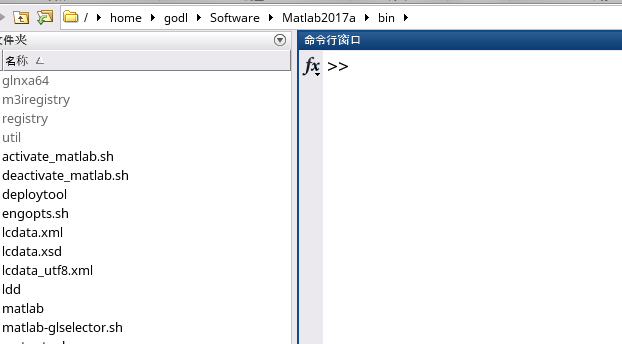点击右上角带绿色箭头的小文件夹,设置当前程序所要存放的路径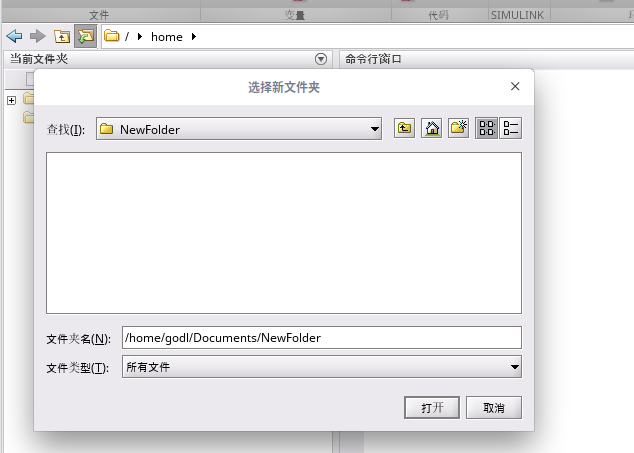点击右上角的新建,选择函数,在下图输入所创建的子函数,然后保存.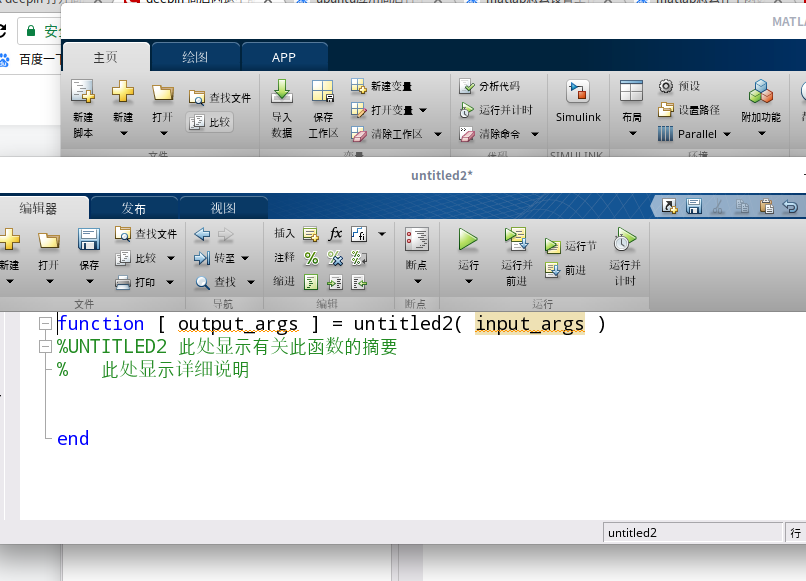输入程序: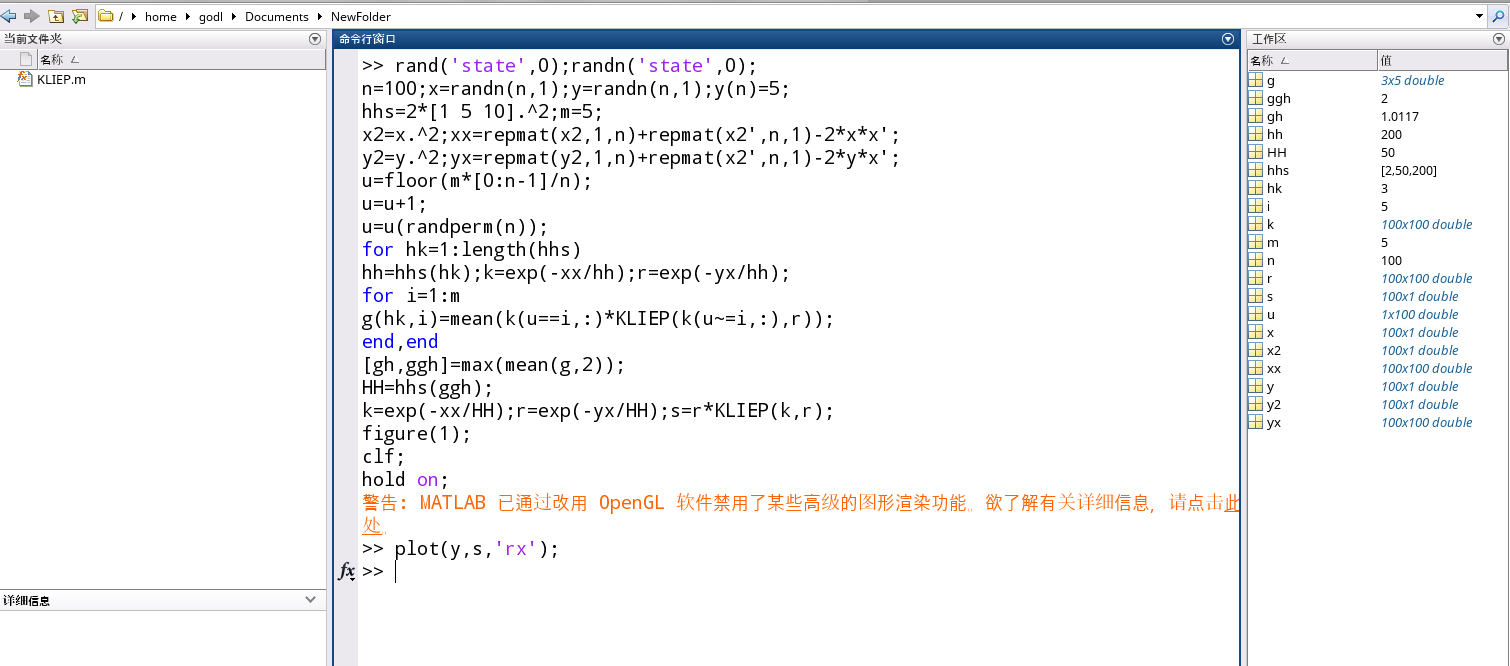结果正确: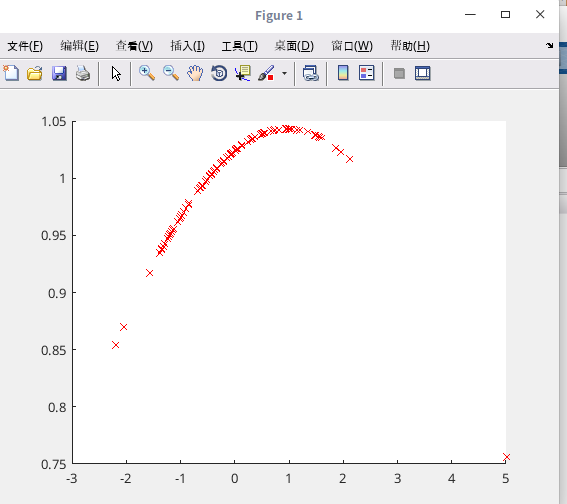展开全文• 本文主要介绍： 1、利用global全局变量将主程序参数传递给子函数。 2、如何在一个文件中定义多个子函数学习 开发语言
• 函数调用在一个m文件中，可以定义多个函数，但是文件名一定要与第一个函数(主函数)一致，该文件中其他函数则为本文件的私有函数，外部不可访问(可以通过参数调用的方法访问，下面会描述)。例如在一个程序中，创建一...
• 本文主要是关于matlab基本函数的使用说明，相信对于初学者是有很大帮助的！
• 对于文件夹中的函数我们需要在程序调用前添加其路径，路径分为绝对路径和相对路径，方便起见写相对路径，值得注意的是我们有时需要修改文件夹的名称或是删除了某些函数，这时我们需要删除已添加路径，以免matlab...
• 2函数文件+子函数定义一个具有多个自定义函数的M文件 ?3Inline:无需M文件直接定义 ?4Syms+subs?无需M文件,直接定义 ?5字符串+subs无需M文件,直接定义. 6匿名函数 7直接通过@符号定义. 1函数文件+调用函数文件定义多...
• 最近笔者学习MATLAB，今天写函数调用matlab的调用其它函数的 变量可以是 全局变量 也可以是通过参数的调用来实现数据的传递。 例如 全局变量 例如 函数的参数传递
• 函数调用Matlab中，调用函数的形式如下： [输出参数 1，输出参数 2，…] = 函数名字（输入参数 1，输入参数 2，输入参数 3，…） 注意事项： 函数调用的时候，参数顺序应该与定义的时候一样。 函数可以嵌套 函数...callback
• 定义anonymous function时,完全定义函数are stored所需的变量：By using anonymous functions, you can also capture certain variables and their values from the function workspace and store them in the ...
• MATLAB中的m文件如何调用子函数刚接触MATLAB不是很懂摸索了半天也不知所以然，，求帮忙这是主函数function fm(a,fm,fc,fs,dt,snr)%本程序实现信号的频率调制%a是调频信号的幅度，fc为载波频率，fm为调制信号频率，fs...
• Matlab中一个函数调用另外一个函数的操作方法方法一：主函数调用子函数首先在Matlab中新建一个m文件函数 Untitled2()，Untitled2()为主函数，然后在函数下方添加一个子函数count(i)，主函数中调用子函数，代码如下：...
• Matlab中一个函数调用另外一个函数的操作步骤作者:小安 来源:PC下载网时间:2019-11-18 13:27:35最近很多伙伴才刚刚安装入手Matlab这款软件，而本节就重点介绍了关于Matlab中一个函数调用另外一个函数的操作步骤，还...
• 2、函数文件+子函数：定义一个具有多个自定义函数的M文件。3、Inline:无需M文件，直接定义。4、匿名函数。5、Syms+subs:无需M文件,直接定义。6、字符串+subs：无需M文件,直接定义。7、直接通过@符号定义...
• Matlab子函数定义在matlab的函数定义中，如果函数如果函数较长或较多，往往可以将各函数分别写在多个.m文件中，但有时函数可能很短，就几行，可能希望将多个函数定义放在同一个.m文件中，这里就存在一个子函数的定义...
• Matlab函数、子函数的定义方法除了最常使用的m文件函数，matlab还可以通过内联函数和匿名函数自定义函数。1. m文件函数以function关键字开头的m文件，并且通常函数名要与文件名相同。例如下面的内容保存为myfun2.m即...
• 答：一般子函数调用，都有输入变量，再返回一个或多个变量。如：fuction [a b]=imag(m,n,p); 这个函数的意思是，输入m n p三个参量，返回a b这两个参量，可以是矩阵 数值 向量 结构体，根据自己的需要来。在调用的...
• 但是，有时，您只需在单个 m 文件中编写一些函数， 尽管只能调用顶部以外的函数在 m 文件中。 这完全没问题，因为您通常期望这些函数永远不会被其他 m 文件使用。 然后，稍后，您发现自己想要访问那些小功能来自...
• 1. 高手指点一下Matlab的主函数和子函数的关系,怎么建立子函数主函数在结构上与其他函数没有一点区别，之所以称之为主函数，就是因为他在M文件上坐了第一把交椅，其他函数都排在他后面。按惯例他与M文件同名，在命令...
• 函数挪用在一个m文件中，可以界说多个函数，但是文件名必定要与第一个函数(主函数)一概，该文件中其他函数则为本文件的私有函数，外部不行访问(可以经历参数挪用的要领访问，下面会形容)。比如在一个步骤中，创建一...
• Matlab函数定义和调用 Matlab 自定义函数的五种方式 命令文件/函数文件+ 函数文件 多个 M 文件 自定义函数的五种方式 函数文件 + 子函数 一个 M 文件 inline 无需 M 文件 符号表达式 + subs 方式 无需 M 文件 字符串...
• % ga函数 options = gaoptimset('Generations', 200,... %设置迭代次数 'PopulationSize', 50,... % 设置种群大小 'UseParallel', true,... % 是否并行 'Vectorized', 'on'); % 指定函数是否向量化 [bestchrom,~,...
• 点击查看matlab中，怎么样用function自定义函数调用另一个函数名为输入？具体信息答：test定义两个参数，一个是函数，一个是函数的变量。 function [z]=test11(funname,x) z=funname(x); 调用方式如下 test11(@bestU...
• 函数与子函数 函数语法结构 函数同其它高级语言一样，是MATLAB语言的重要组成部分，MATLAB除了内部函数外，还有各种工具箱中的函数，这些函数都以M文件给出，以便调用。 下面以一个例子来说明MATLAB函数的语法。......

# matlab子函数调用matlab 订阅